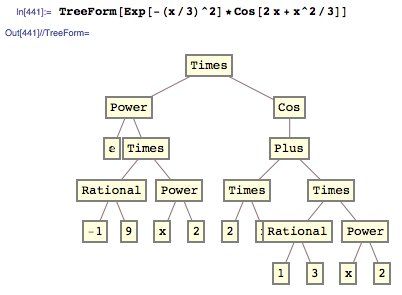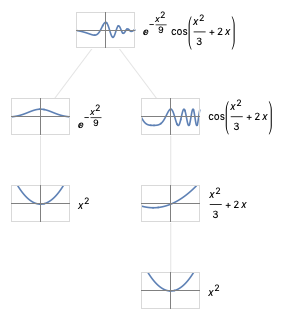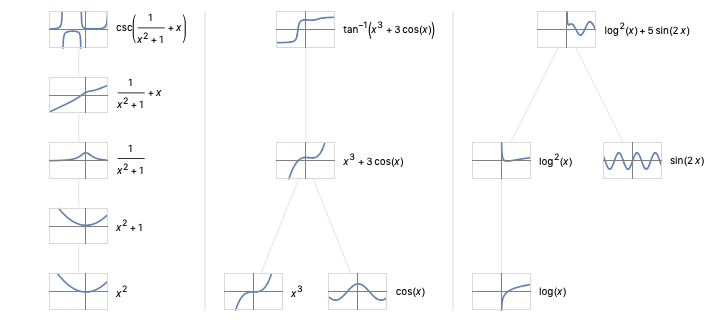I’ve been dwelling on the kinds of intuitions developed by anyone who studies mathematics. They’re often simple rules of thumbs and ways of thinking about mathematical objects. In fact, they are so obvious that once you’ve internalized them, it often doesn’t occur to you to articulate them again.

For example, one very basic component of real analysis is function composition, something that is probably taught early on in high school — although technically I wouldn’t know.

What does it mean to compose functions? How does one reason about a compound function? I got thinking about how one might go about helping a student to develop their intuition about these questions. It occurred to me that there is a simple visualization technique that answers this exact question without any words at all.

How does this work? Let’s say you want to visualize the following compound function:

$$e^{-x^2/9} \cos ( {x^2}/3 + 2x )$$

First, let’s consider the syntax tree of this expression. This is a tree in which the root is the entire expression and the leaves are linear or constant functions like x and 1/3. Luckily, with Mathematica it’s pretty easy to present an arbitrary expression directly in this tree representation by using the formatting construct TreeForm:My idea is to produce actual function plots for each interesting node in this tree. By moving up the tree we can show how these sub-expressions fit together to compose the entire expression. It turns out that it takes about 15 lines of Mathematica to compile the syntax tree, recognize and extract the interesting nodes, and synthesize the corresponding plots into a graphical diagram.

Wrapping this all up into a function called FunctionTreePlot, we can now visualize our example like so:This technique seems to work quite well. You can easily chase visual features of the corresponding plots up and down the tree to answer questions like “why does this function have a pole here” or “what will be the effect of changing this co-efficient?” I hope this functionality can make it into Wolfram|Alpha’s already strong support for visualizing mathematical functions.

Here are a few more examples from my experiments: